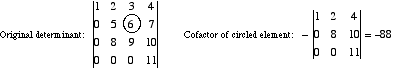index: click on a letter A B C D E F G H I J K L M N O P Q R S T U V W X Y Z A to Z index index: subject areas numbers & symbols sets, logic, proofs geometry algebra trigonometry advanced algebra & pre-calculus calculus advanced topics probability & statistics real world applications multimedia entrieswww.mathwords.com about mathwords website feedback

 Cofactor The determinant obtained by deleting the row and column of a given element of a matrix or determinant. The cofactor is preceded by a + or – sign depending whether the element is in a + or – position.See also